How Cheenta works to ensure student success?
Explore the Back-Story

# RMO 2019 Problems and Solutions | Previous Year QuestionsThis post will provide you all the RMO (Regional Mathematics Olympiad) 2019 problems and solutions. You may find some solutions with hints too.

RMO 2019, Problem 1:

Suppose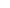is a nonzero real number such that both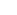and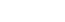are rational numbers. Prove thatis a rational number.

RMO 2019, Problem 2:

Let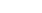be a triangle with circumcircle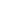and let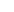be the centroid of triangle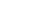Extend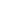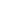andto meet the circleagain in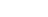and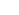, respectively. Suppose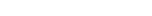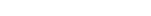and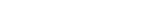Prove thatand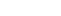are equilateral triangles.

RMO 2019, Problem 3:

Let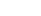be positive real numbers such that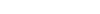. Prove that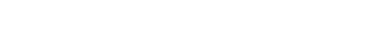RMO 2019, Problem 4:

Consider the following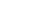array formed by using the numbers 1,2,3,4,5,6: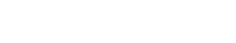RMO 2019, Problem 5:

In an acute angled triangle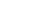let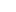be the orthocenter, and let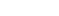be the feet of altitudes from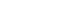to the opposite sides, respectively. Let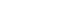be midpoints of segments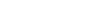respectively. Let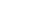be feet of altitudes from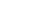on to the line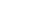. Prove that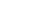is perpendicular to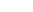.

RMO 2019, Problem 6:

Suppose 91 distinct positive integers greater than 1 are given such that there are at least 456 pairs among them which are relatively prime. Show that one can find four integers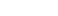among them such that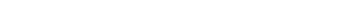.

This post will provide you all the RMO (Regional Mathematics Olympiad) 2019 problems and solutions. You may find some solutions with hints too.

RMO 2019, Problem 1:

Supposeis a nonzero real number such that bothandare rational numbers. Prove thatis a rational number.

RMO 2019, Problem 2:

Letbe a triangle with circumcircleand letbe the centroid of triangleExtendandto meet the circleagain inand, respectively. SupposeandProve thatandare equilateral triangles.

RMO 2019, Problem 3:

Letbe positive real numbers such that. Prove thatRMO 2019, Problem 4:

Consider the followingarray formed by using the numbers 1,2,3,4,5,6:RMO 2019, Problem 5:

In an acute angled triangleletbe the orthocenter, and letbe the feet of altitudes fromto the opposite sides, respectively. Letbe midpoints of segmentsrespectively. Letbe feet of altitudes fromon to the line. Prove thatis perpendicular to.

RMO 2019, Problem 6:

Suppose 91 distinct positive integers greater than 1 are given such that there are at least 456 pairs among them which are relatively prime. Show that one can find four integersamong them such that.

This site uses Akismet to reduce spam. Learn how your comment data is processed.

### Knowledge Partner